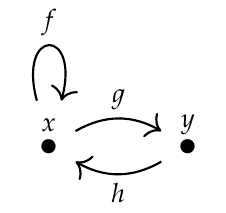Christopher:

> Yeah, I'm trying to remember what I know about raising a matrix to a power. Oh, the natural number powers of a matrix of natural numbers are again natural numbered matrices. So we can then reinterpret them as a graph. Which means given a pointed graph that generates a sequence, we can construct a graph that generates every nth value of the series. Which is pretty non obvious I think!

It becomes obvious if you take a small example, like the matrix

$\left( \begin{array}{cc} 1 & 1 \\\ 1 & 0 \end{array} \right)$

coming from this graphand square, cube, etc. the matrix while also counting paths of length 2, 3, etc. by hand. You'll see that the formula for matrix multiplication

$(AB)\_{ij} =\sum_k A\_{ik} B\_{kj}$

is exactly what you need for path counting, because the right-hand side says "to count the number of ways to go from node \$$j\$$ to node \$$i\$$, sum over all ways to go first from \$$j\$$ to some other node \$$k\$$ and then from \$$k\$$ to \$$i\$$".

There are few things so good for understanding math as actually multiplying matrices by hand.After working through this example for half an hour, it will seem so obvious that you'll realize this is exactly one of the things matrix multiplication was made for!

Heisenberg reinvented matrix multiplication when developing quantum mechanics, for more or less the same reason. Back then, in the 1920's, even good physicists didn't all know about matrices! So Heisenberg reinvented matrix multiplication. Then he told his thesis advisor, Max Born, who said something like "that's just matrix multiplication - read this book".

And thus [matrix mechanics](https://www.google.com/search?q=matrix+mechanics&ie=utf-8&oe=utf-8&client=firefox-b-1) was born. Only much later did it become clear what was really going on: an interesting relation between quantum mechanics and category theory!